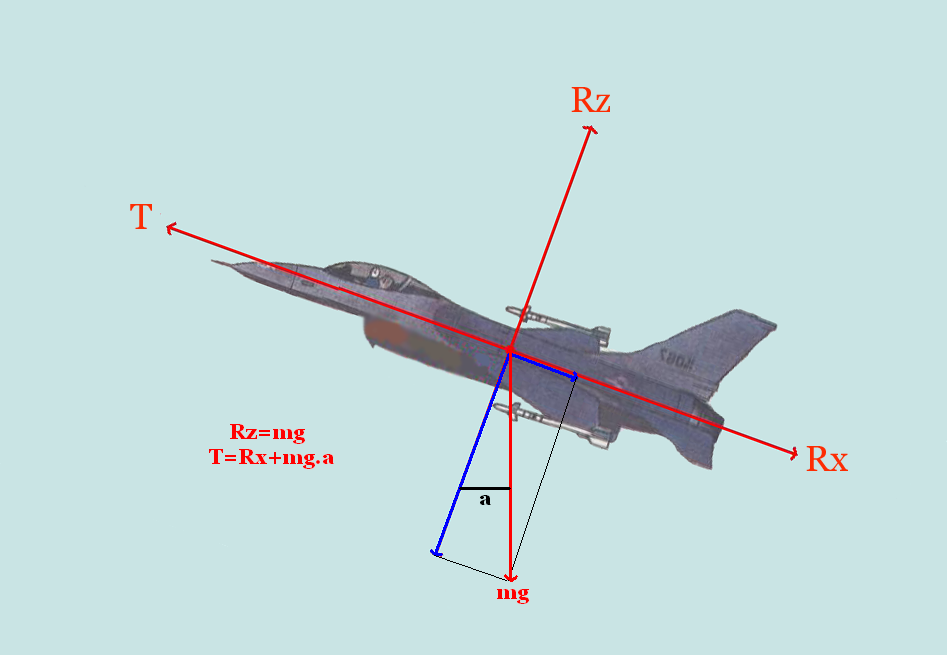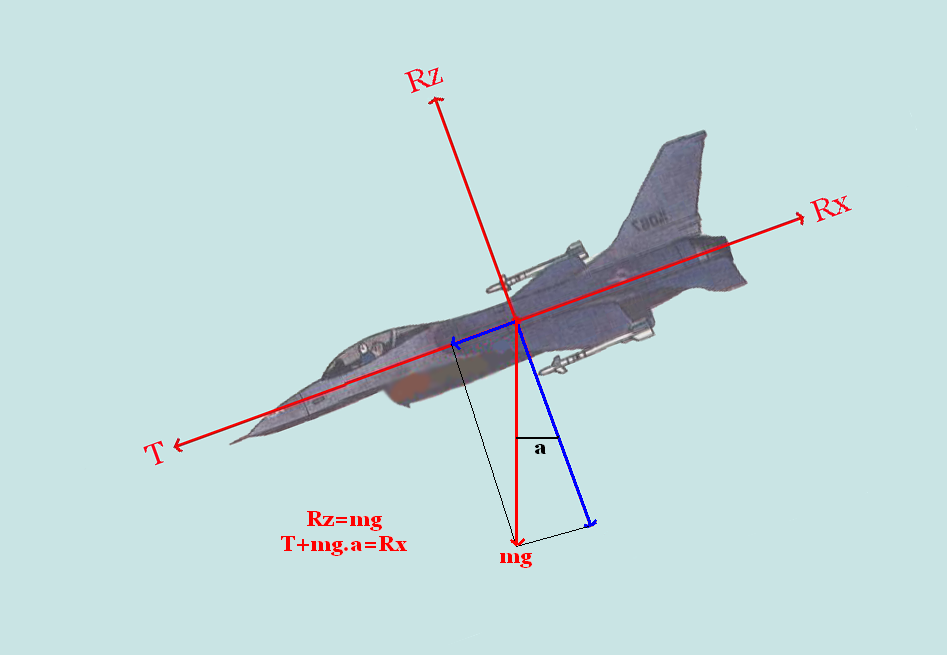# Different kind of flights

## I. The various types of flight.

### A. The flight in uniform rectilinear landing

Let us begin with the simplest case of the flight of a plane: the flight in uniform rectilinear landing. Here is first of all a reminder of the plan of four strengths being applied on a plane in this configuration, these strengths are named as wants it the international system :

The strengths balance each other here two-two:Rz = mg and T = Rx

### B. The flight in uniform rectilinear ascent/rise ?

So that a plane rises, the portance has to be this time superior to the weight, logical, but it is also necessary that the strength of push or drive exercised by one or several engine(s) or superior to that of the flight in landing, because when the plane rises, the trail has to make stronger. In ascent/rise ??, as in descent moreover, we can decompose the weight of the plane into two strengths: the one parallel to the trajectory of the plane, going towards the back, the other one perpendicular in this trajectory, and going downward. The real weight is the resultant of these two strengths. Let us suppose now that the plane rises by making a noted angle "a" with the horizontal. We can calculate the portance, as well as the push / necessary drive to make rise the plane, according to this angle of rise/ascent ?? The calculations are the following ones:

Rz = mg.cos has and T = Rx + mg.sin has.

A plane rises generally on a rather low slope, we thus consider that cos a = 1, and that sin a = tan a. We can thus return, in the case of a normal rise/ascent ??, the previous equations to these:
Rz = mg and T = Rx + mg.a.
What allows to express has for this: a = ( T-Rx) / mg.
The slope of rise/ascent is noted in %, it is thus equal to 100. ((T-Rx) / mg).
A plan while bringing to light the strengths and resultant which are applied during the rise/ascent :We can also calculate the upward speed of the plane, that is the speed in which it climbs on a vertical axis, this speed is noted Vz and is expressed in meters per second, she obtains by this calculation:

Vz = V. ((T-Rx) / mg) V being the speed of the plane in meters per second.

### C. The flight in uniform rectilinear descent

The configuration of the flight in uniform rectilinear descent is not very different from that of the flight in uniform rectilinear rise/ascent ??. The portance does not decrease, but the power of engine(s) is reduced. "a" always indicates the angle that make the plane with its horizontal axis, the speed of the plane is constant on its trajectory. The major change here is that the constituent of the weight which is parallel to the trajectory goes to the same direction as the strength of drive / propulsion. The calculations serving to determine the portance and the push / drive during a descent are the following ones:
Rz = mg.cos has and T + mg.sin has = Rx.We always consider for the same reasons as in a rise/ascent??, as cos a = 1 and as sin a = tan a. The equations spell then:

Rz = mg and T + mg.a = Rx,

What mean saying that :

A = (Rz - T) / mg.
The slope of descent, noted in % as for the rise/ascent??, is noted 100. ((Rx - T) / mg).

We can calculate the vertical speed of descent, otherwise called rate of fall, and noted Vz, as for the upward speed: Vz = V. ((Rz - T) / mg). The meter per second is the reference unit.

### D. The flight in symmetric bend in landing

Here is a configuration very different from the flight in descent, in rise/ascent?? and in landing, the flight in bend put at stake a strength which allows it to turn, this strength influences the portance. We say that the bend is symmetric when the vector speed of the plane stays in the axis of flight of the plane. In our case, the speed is constant and, I have to call back(remind) it, horizontal.

The balance of the strengths is more complex here, the portance indeed has to be superior to the weight so that the balance is made, because of the slope of the plane, the increase of the portance also allows to keep/preserve ??? the speed which the plane had before the bend, only, for it the pilot pulls slightly the handle towards him to increase the incidence of the plane, what also has the effect of increasing the trail. It is thus necessary to compensate by increasing slightly the engines' power.
The flight in bend stages a new report that one that we name factor of load, it is noted "n" in the international system: n = Rz / mg. The factor of load depends on the slope, in consequent, we can calculate it according to the angle of bend by this calculation:

N = 1 / cos a (has being the angle of inclination in degree).
The factor of load is rather known, it is in fact about famous " G's " which the fighter pilots or acrobatics take when they are in action. 1 G corresponds to the weight of the considered mobile, if the pilot cashes 3 Of the G, it weighs 3 times its weight. Here is the plan illustrating the flight in symmetric bend in landing:It is possible that the bend is not symmetric, it is then slipped. At this moment there, the properties which we saw do not apply.
This last one represents the balance between all the aerodynamic strengths for a given speed. In constant speed the aircraft does not come down nor rises, the vector weight is equal to its opposite the portance, idem for the trail countered by the propulsion.

It is thus applied as strengths on the plane:

1. The weight
2. The portance and the trail
3. The drive

The peculiarity of the landing, compared with the rise/ascent ?and with the descent, is that the portance - perpendicular in the relative wind - is vertical, equal and set against the weight.
The control of the landing is made by keeping a plate shown by means of outside references, or of the artificial horizon.

4 votes. Average: 2.75 / 5.

Make a free website with emyspot.com - Report abuse